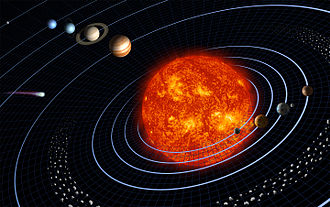# Introduction to Energy

## Energy

• We use the symbol E to represent energy.
• The concept of energy is one of the most important topics in science.
• Every physical process that occurs in the Universe involves energy and energy transfers or transformations.
• Energy is defined as the measure of a system's ability to do work.
• Energy is conserved; it can not be created or destroyed; it can be transformed from one form to another; however, the total amount of energy remains the same.
• Energy has the same units as Work: Joule (J).
There are various types of energy. For now, we will focus on two types of Mechanical Energy (energy due to movement and position):
• Kinetic energy (KE)is the energy associated with an object’s motion. The formula of KE is experessed as ½mv2
where m is the object's mass and v is the object's speed
• Potential energy (PE) is the energy associated with the system’s position or orientation.
Gravitational Potential Energy (PE) is an energy stored in an object due to its position (height); it equals the work done by gravity. PE = W = Fd
Conservative forces are forces for which the energy can be completely recovered.
Gravity and elastic forces are conservative. But friction is not conservative.

Example 1:
If you lift an object, you must apply a force at least equal to the object's weight. F = mg
Lifting the object through a distance d, the work done is PE = W = Fd = mgd
Therefore, PE = weight × height = mgh

Example 2:
The term potential energy implies storing energy to use later for other purposes.
As the archer exerts force on the bow and draw the string back (see figure below)The (elastic) PE is then released and the force does work on the arrow.
The PE in the bow limbs is transformed into the KE of the arrow.

Example 3:
As illustrate in the figure below, the gravitational force maintains the planets in orbit around the Sun.